## Introduction

Mass transfer phenomena across gas-liquid interfaces are often seen in geophysical and industrial processes, and such mass transfer is believed to be enhanced by wind-driven turbulence (i.e., surface-renewal motions) near the interface on the liquid side (e.g., Jähne et al.1,2,3, Komori et al.4,5, Takagaki et al.6, Kurose et al.7). In order to clarify the mass transfer mechanism and precisely evaluate the amount of the mass transferred across the wind-driven wavy gas-liquid interface, direct numerical simulations (DNSs) of gas-liquid two-phase turbulent flows with wind-driven wavy interfaces were carried out by some researchers (Komori et al.5, Takagaki et al.6, Kunugi et al.8, Lakehal et al.9,10,11, Banerjee12, Banerjee et al.13). One of the most important properties to globally predict the amount of mass transferred across such gas-liquid interfaces is the mass transfer coefficient, kL, and therefore a precise model for it is necessary. Here, kL is defined as: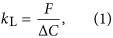where F is the mass flux at the gas-liquid interface per unit area, ΔC the mass concentration difference between the interface and the bulk liquid. The value of kL is often correlated with the Schmidt number, Sc (=νL/DL), on the liquid side, where νL and DL are the kinematic viscosity and molecular diffusivity on the liquid side, respectively. However, the evaluations of kL are limited for Sc ≤ 1600 in previous experiments (e.g., Jähne et al.1,2,3, Komori et al.4, Liss14, Broecker et al.15, Wanninkhof16, Iwano et al.17,18) and limited for Sc ≤ 100 in previous numerical simulations (e.g., Komori et al.5, Takagaki et al.6, Banerjee et al.13). Also, since the flow and mass conditions are different among these previous experimental and numerical studies, the universal relation between kL and Sc has not been clarified yet, even in these Sc ranges.

In this paper, we aim to present the relationship between kL and Sc in the wide range of 0.7 ≤ Sc ≤ 1000 by applying a SEMI-DNS, in which the mass transfer is solved based on an approximated deconvolution model (ADM) scheme proposed by Stolz and Adams19, to a gas-liquid two-phase turbulent flow with a wind-driven wavy interface.

## Methods

The numerical procedure of the DNS used here was the same as in the study by Komori et al.5 and Takagaki et al.6. In the procedure, the wind-driven wavy gas-liquid interface was captured by the arbitrary Lagrangian-Eulerian (ALE) method with boundary-fitted coordinates (BFC) on moving grids (Komori et al.5,20, Takagaki et al.6, Lakehal et al.9,10,11, Banerjee et al.13, Fulgosi et al.21, Lin et al.22, Guo and Shen23, Tsai et al.24). The non-dimensional governing equations for an incompressible Newtonian fluid flow with mass transfer are given by the equation of continuity, Navier-Stokes (N-S) equation, and transport equations of mass using the Einstein summation convention: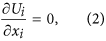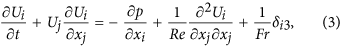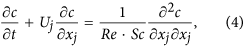where Ui is the i th component of the velocity vector (i = 1, 2, and 3 denote the streamwise, spanwise and vertical directions, respectively), p the pressure, δij the Kronecker’s delta, and c the mass concentration. Here, equation (4) is solved only on the liquid side. The non-dimensional parameters, Reynolds number Re, Schmidt number Sc and Froude number Fr are defined as: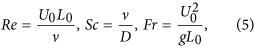where L0 and U0 are the reference length and velocity, respectively, ν the kinematic viscosity, DL the molecular diffusivity of mass on the liquid side, and g the acceleration due to gravity. On the gas-liquid interface, two boundary conditions should be satisfied. One is the kinematic boundary condition that describes the Lagrangian behavior of the fluid particle on the mobile gas-liquid interface, and the other is the dynamic boundary condition, which is determined from the balance of stresses acting on the gas-liquid interface in the normal and tangential directions (Komori et al.5, Takagaki et al.6).

Since a high Sc mass causes a smaller Batchelor scale (Hasegawa and Kasagi25,26,27, Kurose et al.28,29), the mass transfer was solved based on ADM scheme proposed by Stolz and Adams19 and modified by Mathew et al.30. The model based on DNS of flow field and ADM of mass field is proposed by Schwertfirm and Manhart31 and is called as SEMI-DNS. The ADM method is briefly explained here, and see more details in Schwertfirm and Manhart31. When a mass concentration field, f, is predicted with meshes coarser than the Batchelor scale, the mass concentration field is like a filtered mass concentration field,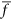. Now, by the filtering function, G, the relation between f andare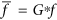, where * is the filtering procedure. On the ADM method, without any LES model, the mass concentration field is predicted using the inverse filtering function, Q. Generally, under the assumption of the existence of the inverse matrix, G−1, the Taylor expansion of G−1 is shown as: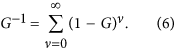By equation (6), Stolz and Adams19 defines Q as: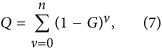and, therefore, Q has the property of inverse matrix, that is, Q*G = G*Q ~ I and Q ~ G−1. Therefore,can be restored by Q shown as: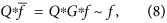where they defines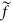as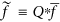. In this study, the simple filtering shown as: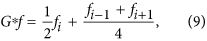is used. Uisng equation (8), equation (4) is rewritten (see the details in Schwertfirm and Manhart31) as: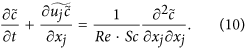The computational domain was 8δ × 3.92δ × 3δ (δ = 1.25 × 10−2 m) in the streamwise (x), spanwise (y) and vertical (z) directions. The origin (x = y = z = 0) was located at the height of 2δ from the bottom, and the initial flat gas-liquid interface which divides the two-phase flow between upper gas and lower liquid streams was placed on the plane of z = 0. The grid points used in the streamwise (x), spanwise (y) and vertical (z) directions were 200 × 98 × 60 on the gas side and 200 × 98 × 120 on the liquid side, respectively. The grid spacing was equidistant in the streamwise (x) and spanwise (y) directions, and to get high resolution the nonuniform meshes clustered in the gas-liquid-interface region were used in the vertical (z) direction. Periodic boundary conditions were applied in the streamwise (x) and spanwise (y) directions, and slip boundary conditions were applied at the top and bottom boundaries. As initial conditions of flow field, a fully developed wall turbulent flow and a quiescent flow were given on the gas and liquid sides of the initial flat interface, respectively. For the computation of the mass transfer on the liquid side, the boundary conditions of the passive mass at the gas-liquid interface and the bottom boundary were given by C = 1.0 and Neumann condition, respectively. The marker and cell (MAC) (Harlow and Welch32) method was used to solve the Navier-Stokes equations. In order to induce the realistic deformation of the gas-liquid interface, a fully developed wall turbulence for an initial free stream wind speed of U∞,ini = 4.8 m/s and an initial friction velocity of u*,ini = 0.242 m/s was given on the gas side over a flat quiescent liquid. The gas flow was driven by pressure gradient imposed in the streamwise direction. The Reynolds number based on U∞,ini and δ, Reini, was 3960 and that based on u*,ini and δ, Re*ini, was 200. The density ratio of the gas and liquid was 830, which corresponds to the value for the air-water two phase flow at about 20 °C. The Schmidt number, Sc, on the liquid side ranged from 0.7 to 1000 (11 cases). For comparison, the computations for a forced flat gas-liquid interface were also carried out in the same conditions as for the wind-driven wavy gas-liquid interface (11 cases). Each computation was performed until the flow field on the liquid side reaches statistically steady state. The CPU times for the wind-driven wavy and flat interfaces were about 3900 hours (10 days of wall-clock time using 16 cores) and 5200 hours (14 days of wall-clock time using 16 cores) for 2,400,000 steps and 6,400,000 steps (6.0 seconds and 16.0 seconds) on the super computer NEC:SX-ACE, respectively. Figure 1 shows the instantaneous configuration of the wind-driven gas-liquid interface at a fully-developed time.

## Results and Discussion

The mass transfer coefficient on the liquid side, kL, (Eq. (1)) is calculated as: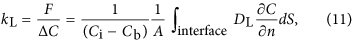where ΔC = Ci − Cb (here, Ci and Cb are the mass concentrations of the interface and bulk liquid, respectively, and Cb is set to be zero in this study), n the normal direction with respect to the gas-liquid interface, A the surface area of the interface, and DL the molecular diffusivity of the mass on the liquid side. For both the wind-driven wavy and flat interfaces, the statistics were taken after the flows fully develop and the values of kL indicate statistically steady values. That is, in the wind-driven wavy interface, we started the wind-water simulation with initial flat air-water interface, defined as t = 0 s. We started to solve the mass concentration field also at t = 0 s. We calculated a time-averaged kL by use of the the mass concentration field during the time period t = 5.0 s to 6.0 s. In flat interface condition, we similarly started the wind-water simulation at t = 0 s, but we did not start to solve the mass concentration field until t = 10 s, and we calculated a time-averaged kL by use of the mass concentration field from t = 15.0 s to 16.0 s. The statistics of wind and waves are also taken in the same manner as Komori et al.5 and Takagaki et al.6, and the values are listed in Table 1. Here, the uniform velocity on the gas side, U, is defined as the velocity on the upper wall of the computational domain. The wind speed at 10 m height above the gas-liquid interface, U10N, drag coefficient, CDN, and surface current, USURF, are estimated in the same manner as Komori et al.5 and Takagaki et al.6. Each wind wave is determined by applying the zero-up cross method to the spatial fluctuation of the water level. First of all, we obtained the streamwise distribution of surface elevation (Fig. 2). Using the zero-up cross method, we detected locations with zero-up cross (see positions A, B, and C in Fig. 2). Then, for example, a wave is defined as being in the area between positions of A and B in Fig. 2. From those waves, we selected the largest one-third waves, and defined the significant waves as the largest one-third waves. The significant wave height, HS, and significant wave length, LS, are defined as the mean wave height and length, respectively, for the largest one-third waves. The phase speed of the significant wind-waves, Cp, is measured by analyzing the propagation of the significant wind-waves.

Figure 3 shows the relationship between distributions of instantaneous local mass flux on the gas-liquid interface and the mass concentration on the liquid side (y-z and z-x planes) for the wind-driven wavy interface for Sc = 1.0 and 1000 at t = 5.0 s. Here, the local mass flux, Flocal, on the gas-liquid interface is estimated by: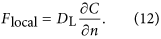It should be noted that the color ranges of Flocal and the mass concentration for Sc = 1.0 and 1000 are different in this figure. The distributions of Flocal and the mass concentration on the liquid side for Sc = 1.0 and 1000 are observed to be similar, namely, streaky motions of the mass flux on the gas-liquid interface are strongly associated with the streamwise vortices related to downward bursting motions appearing beneath the interface. The mass transfer mechanism across the wind-driven wavy interface is illustrated in Figure 24 in Komori et al.5. In summary, a pair of streamwise vortices causes downward bursting motions beneath the streaky regions with both low-mass flux and high streamwise velocity of the gas-liquid interface, and the peeling process happens between the downward bursting motions. Due to this process, the surface layer thickness is reduced and the gradient of the mass concentration increases, and then the mass flux is enhanced. It is evident that the distribution of Flocal is streaky and the amount of the mass entrained into the liquid side is lower for Sc = 1000 than those for Sc = 1.0 due to the lower diffusivity, although the locations of the low-Flocal streaks on the gas-liquid interface are identical between them. It is also confirmed, from the figure, that high Sc mass (Sc = 1000) induces a smaller scale structure than low Sc mass (Sc = 1.0).

Figure 4 shows the relationship between the mass transfer coefficient, kL+, normalized by the friction velocity on the liquid side and Sc, together with those obtained by previous experiments (Jähne et al.3, Iwano et al.17,18, Hanratty33) and computations for the wind-driven wavy interface (Komori et al.5, Takagaki et al.6, Banerjee et al.13) and the flat interface (Hasegawa and Kasagi27,34,35, Calmet and Magnaudet36). Here, Hanratty’s33 range is obtained using the experimental data of Jähne et al.2, Liss14, Broecker et al.15, and Merlivat and Memery37. Similar to the previous studies, the predicted kL+ for both the wind-driven wavy and flat interfaces tend to be proportional to Sc−0.5 (see Jähne et al.1), except in the low Sc range. It is interesting to recognize that the trends of kL+ against Sc are similar between the wind-driven wavy and flat interfaces. This is due to the fact that the mass transfer across the gas-liquid interface is controlled by streamwise vortices on the liquid side even for the wind-driven turbulence under the conditions of low wind velocities without wave breaking (Komori et al.5). On the other hand, there appear discrepancies in kL+ between the present computations for the wind-driven wavy and flat interfaces and between the present computations and the previous experiments for the wind-driven wavy interfaces. The former discrepancies in kL+ predicted by the present computations between the wind-driven wavy and flat interfaces are considered to be due to the presences of the gravity/capillary waves with Langmuir circulations based on the discussions in Komori et al.5 and Takagaki et al.6, in which the mass flux distributions on the gas-liquid interfaces between the wind-driven wavy and flat interfaces are compared. The latter discrepancies in kL+ between the present computations and the previous experiments for the wind-driven wavy interfaces are considered to be due to the presence of surface contamination in the experiments, based on the discussions in Komori et al.4 and Komori and Shimada38. As shown in Fig. 4, the predicted kL+ for the wind-driven wavy interface for Sc = 600 is 100~200% larger than the measured values in Iwano et al.17,18 where the free stream wind speed ranges as U = 3.4~9.5 m/s. Similarly, the present values of kL+ for the wind-driven wavy interface tend to be larger than the measured values in Jähne et al.3 as a whole. These results agree well with the statement in Komori et al.4 and Komori and Shimada38 that the predicted value of kL+ becomes higher than that in experiments due to the presence of surface contamination in the experiments. It also confirmed that the filtering difference (equation (9)) is negligibly small on present results (e.g. Fig. 4).

The surface divergence model is considered as one of the models suitable for estimating the gas-liquid mass transfer coefficients (e.g. Banerjee et al.13, McCready et al.39). McCready et al.39 proposed an empirical model for the mass transfer coefficient on the liquid side, kL,model, as: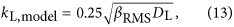where DL is the molecular diffusivity of mass on the liquid side, and βRMS is the root-mean-square (RMS) value of the surface divergence, β. Here, β is defined as: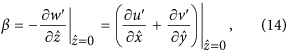where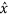and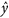are the tangential directions, andis the normal direction. In addition, u′ and v′ are the tangential fluctuating velocities in the streamwise and spanwise directions, respectively, and w′ is the normal fluctuating velocity. The time-averaged RMS values of βRMS are 36.5 s−1 (wind-wave) and 4.6 s−1 (flat). Figure 5 shows the relationship between mass transfer coefficients, kL, and the values kL,model estimated from equations (13). Here, the vector spacings for calculating the surface divergence are 0.5 mm as in the study by Takagaki et al.6, and 0.5~0.7 mm and 0.7 mm in the study by Turney and Banerjee40 on an open-channel gas-liquid interface, and on a wind-sheared gas-liquid interface, respectively. We can see moderately good agreements between our modeled data and the empirical data of previous studies (Turney and Banerjee40). Slight disagreement of equation (13) with experimental and simulated kL exists at low and high Sc, as seen in Fig. 5, and the coefficient of 0.25 is likely to depend on details of the flow field. Turney and Banerjee40 showed that at wind conditions from approximately 4.0 to 10 m/s the capillary waves present in the capillary-gravity mixed wave field will not contribute toward both kL due to dynamics of the advection-diffusion equation. This causes the needs for a combination of concepts from the surface renewal model and surface divergence model40. For the purposes of this present paper, Fig. 5 shows surface divergence is an approximate proxy for gas-liquid mass transfer in a wide range of Schmidt number (0.7 ≤ Sc ≤ 1000).